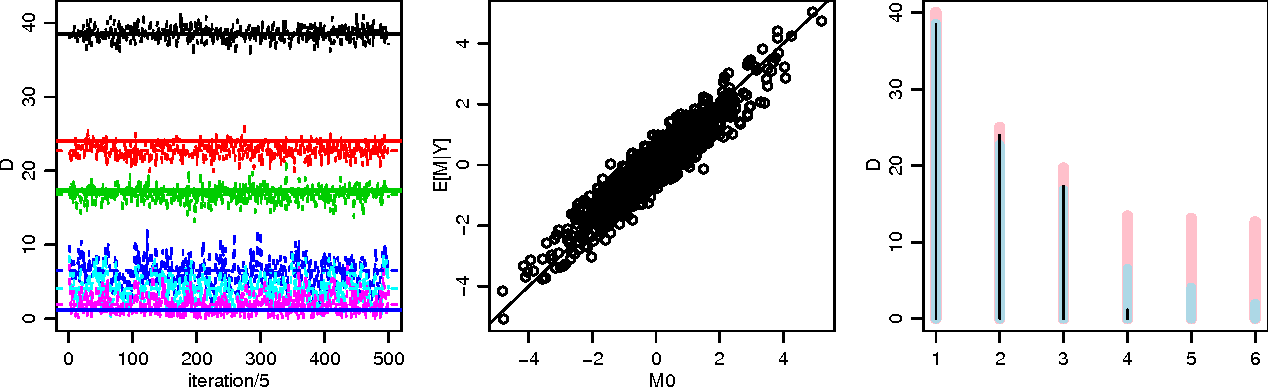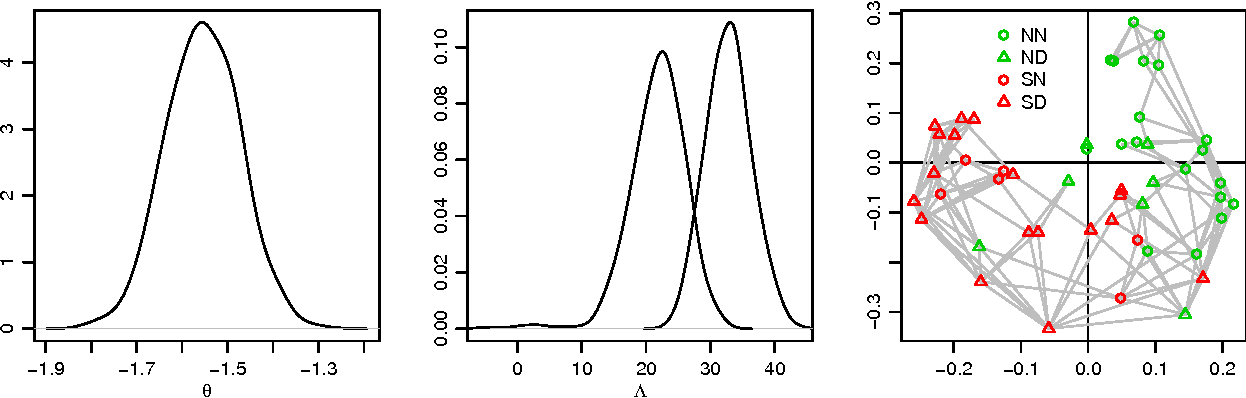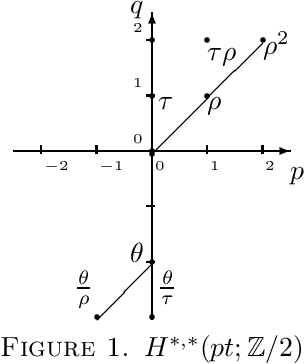You are currently offline. Some features of the site may not work correctly.

# stiefel

National Institutes of Health

## Papers overview

Semantic Scholar uses AI to extract papers important to this topic.
2015
2015
• 2015
• Corpus ID: 119270460
We compute the cohomology of the right generalised projective Stiefel manifolds and use it to find bounds on the rank of the… Expand
Is this relevant?
2013
2013
We illustrate the use of the R-package "rstiefel" for matrix-variate data analysis in the context of two examples. The first… Expand
••Is this relevant?
2011
2011
Abstract In this paper, we compute the RO ( Z / 2 ) -graded equivariant cohomology of rotation groups and Stiefel manifolds with… Expand
•Is this relevant?
2010
2010
Let p be an odd prime, and fix integers m and n such that 0<m<n .p 1/.p 2/. We give a p ‐local homotopy decomposition for the… Expand
Is this relevant?
1979
1979
• 1979
• Corpus ID: 53984749
Stiefel's combinatorial formula for the Stiefel-Whitney homology classes of a smooth manifold is proved, by verifying that the… Expand
Is this relevant?
1973
1973
• 1973
• Corpus ID: 54065197
Introduction. Let Sp(») be the symplectic group and W . = Sp(n)/Sp(n k) the symplectic Stiefel manifold. For k > m one has an… Expand
Is this relevant?
1968
1968
• 1968
• Corpus ID: 6762398
Introduction. Let G be a compact connected Lie group and H a closed subgroup of G. Then the coset space G/H is a smooth manifold… Expand
Is this relevant?
1964
1964

Is this relevant?